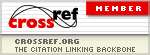### Computing Bond Values to Teach Time Value of Money Principles

Carl B. McGowan, Donald T. Joyner

#### Abstract

The objective of this paper is to demonstrate how to use bond valuation to teach students how to use a financial calculator. In this paper, a single bond issuance is examined using three different yields to maturity (i.e. market rates). The present values of a \$1000 bond issue, valued with five years to maturity and a ten percent coupon rate, are determined using three different yields to maturity: 8% (which would result in a premium), 10% (which would result in neither a discount nor a premium), and 12% (which would result in a discount). The present value of the bond is determined by calculating the present values of both the coupon payment stream (an annuity) and the maturity value (present value of a lump sum) given three different situations. All three values are determined for each year as the time to maturity decreases. A discount (premium) from a change in the YTM is reduced each year until maturity. The results are shown in tables and graphed in Figure 2.

#### Full Text:

PDF

DOI: https://doi.org/10.11114/afa.v1i2.862

### Refbacks

• There are currently no refbacks.

Paper Submission E-mail: afa@redfame.com

Applied Finance and Accounting (AFA)

ISSN 2374-2410(Print)           ISSN 2374-2429(Online)